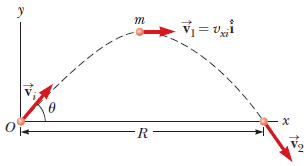# Angular momentum of a particle with a symmetrical path

## Homework Statement

A projectile of mass m is launched with an initial velocity [PLAIN]https://www.webassign.net/images/boldv.gif[I]i[/I] [Broken] making an angle θ with the horizontal as shown below. The projectile moves in the gravitational field of the Earth. Find the angular momentum of the projectile about the origin when the particle is at the following locations. (Use the following as necessary: vi, θ, m, and g for the acceleration due to gravity.)(a) at the origin
(b) at the highest point of its trajectory
(c) just before it hits the ground

L=r x p

## The Attempt at a Solution

For (a) angular momentum is zero because there is no r.

I mean, the problem overall seems easy, but how on earth do I find r for the other two positions? The answer doesn't help with how this is found at all. Is there some sort of property or formula with symmetrical projectile motion?

Last edited by a moderator:

andrewkirk
Homework Helper
Gold Member
Given that you abandoned the last thread you started, not even acknowledging the response, it seems unlikely that anybody would be motivated to help you with this one.

I asked that question and then went to bed. Then I started reviewing every other chapter and have been doing solely that, so I apologize, since that problem had been pretty much the least of my worries.
I was literally planning on replying to that thread once I reached that chapter. This is the chapter prior to that one.

Since the angular momentum depends on then cross product of the position vector and momentum vector(which is in the same direction as velocity),the formulae for the angular momentum maybe written as mvrperpendicular. (The cross product of the component of r vector along/parallel to velocity/momentum is 0)
Find the perpendicular distance of the velocity vector from the origin.for eg in the first case it is 0 since the velocity vector passes through the origin and in the second case it is the max height of the projectile.